Скачать презентацию 1 Теория Информации В. Н. Ярмолик Лекция 2

it_lec_2.ppt

• Размер: 1.7 Mегабайта
• Количество слайдов: 25

## Описание презентации 1 Теория Информации В. Н. Ярмолик Лекция 2 по слайдам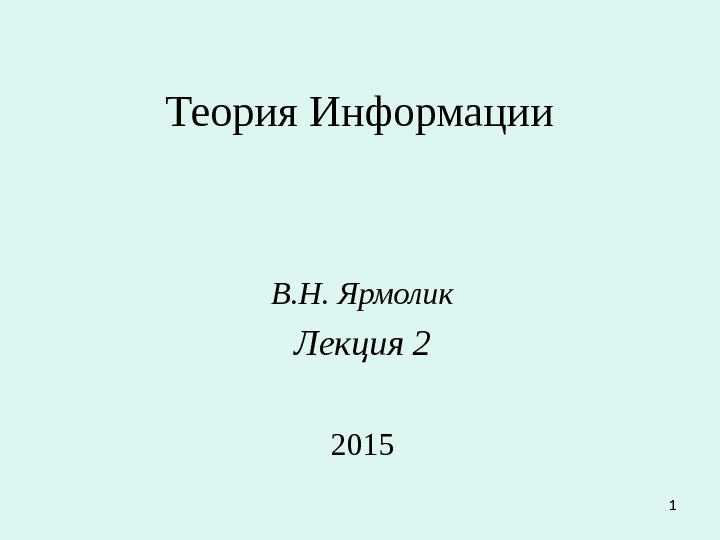1 Теория Информации В. Н. Ярмолик Лекция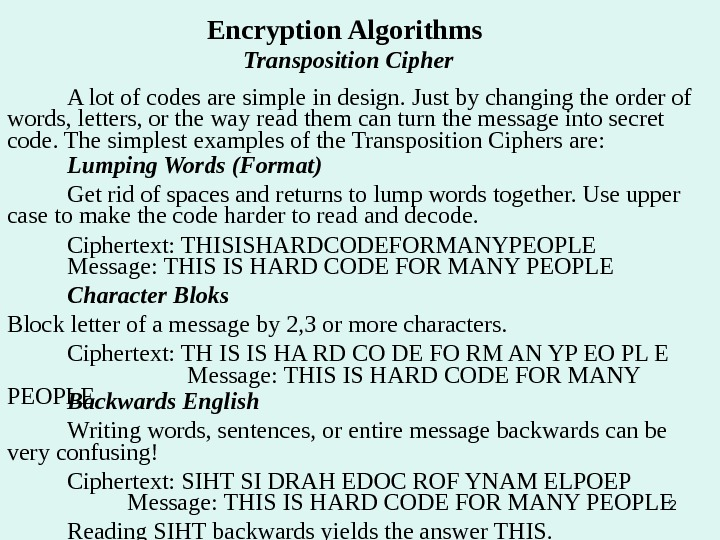2 Encryption Algorithms Transposition Cipher A lot of codes are simple in design. Just by changing the order of words, letters, or the way read them can turn the message into secret code. The simplest examples of the Transposition Ciphers are: Lumping Words (Format) Get rid of spaces and returns to lump words together. Use upper case to make the code harder to read and decode. Ciphertext: THISISHARDCODEFORMANYPEOPLE Message: THIS IS HARD CODE FOR MANY PEOPLE Character Bloks Block letter of a message by 2, 3 or more characters. Ciphertext: TH IS IS HA RD CO DE FO RM AN YP EO PL E Message: THIS IS HARD CODE FOR MANY PEOPLE Backwards English Writing words, sentences, or entire message backwards can be very confusing! Ciphertext: SIHT SI DRAH EDOC ROF YNAM ELPOEP Message: THIS IS HARD CODE FOR MANY PEOPLE Reading SIHT backwards yields the answer THIS.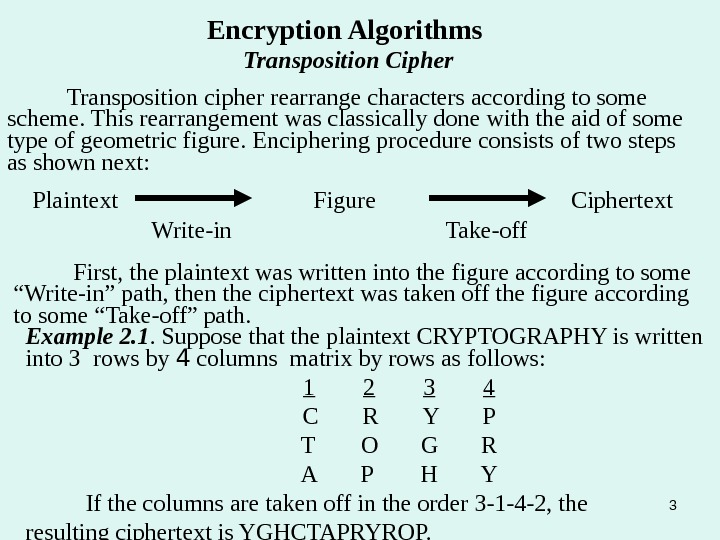3 Encryption Algorithms Transposition Cipher Transposition cipher rearrange characters according to some scheme. This rearrangement was classically done with the aid of some type of geometric figure. Enciphering procedure consists of two steps as shown next: Plaintext Figure Ciphertext Write-in Take-off First, the plaintext was written into the figure according to some “Write-in” path, then the ciphertext was taken off the figure according to some “Take-off” path. Example 2. 1. Suppose that the plaintext CRYPTOGRAPHY is written into 3 rows by columns matrix by rows as follows: 1 2 3 4 C R Y P T O G R A P H Y If the columns are taken off in the order 3 -1 -4 -2, the resulting ciphertext is YGHCTAPRYROP.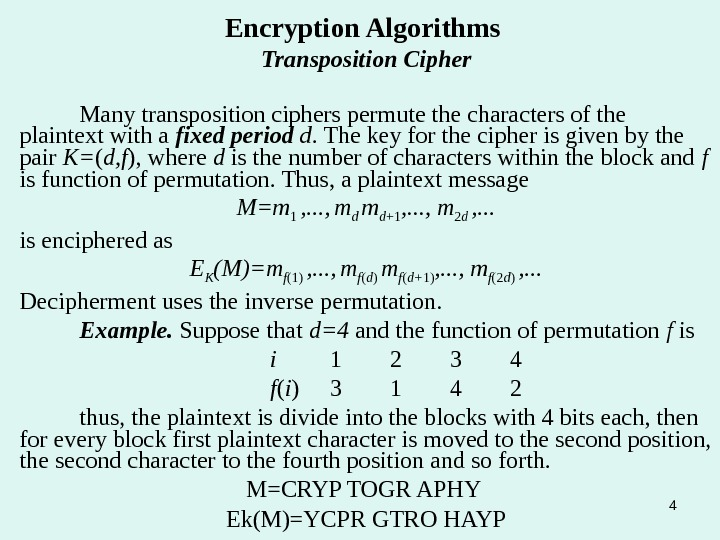4 Encryption Algorithms Transposition Cipher Many transposition ciphers permute the characters of the plaintext with a fixed period d. The key for the cipher is given by the pair K= ( d, f ), where d is the number of characters within the block and f is function of permutation. Thus, a plaintext message M=m 1 , . . . , m d +1 , . . . , m 2 d , . . . is enciphered as E K (M)=m f (1) , . . . , m f ( d ) m f ( d+ 1) , . . . , m f (2 d ) , . . . Decipherment uses the inverse permutation. Example. Suppose that d=4 and the function of permutation f is i 1 2 3 4 f ( i ) 3 1 4 2 thus, the plaintext is divide into the blocks with 4 bits each, then for every block first plaintext character is moved to the second position, the second character to the fourth position and so forth. M=CRYP TOGR APHY Ek(M)=YCPR GTRO HAYP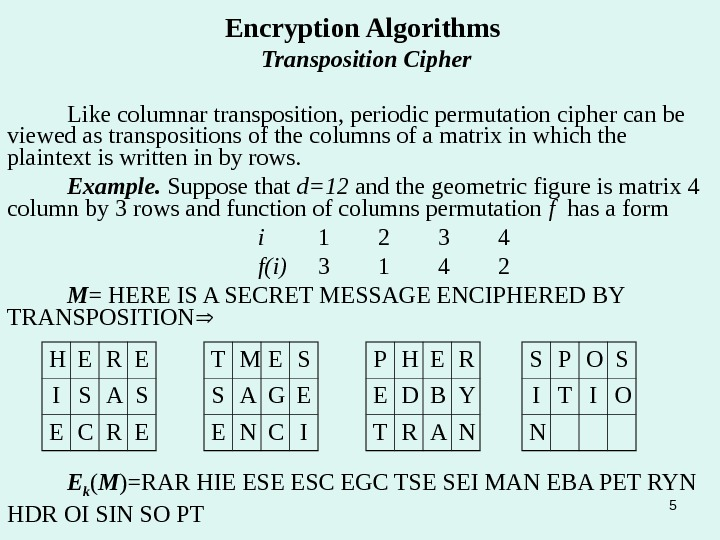5 Encryption Algorithms Transposition Cipher Like columnar transposition, periodic permutation cipher can be viewed as transpositions of the columns of a matrix in which the plaintext is written in by rows. Example. Suppose that d=12 and the geometric figure is matrix 4 column by 3 rows and function of columns permutation f has a form i 1 2 3 4 f(i) 3 1 4 2 M = HERE IS A SECRET MESSAGE ENCIPHERED BY TRANSPOSITION H E R E I S A S E C R E T M E S S A G E E N C I P H E R E D B Y T R A N S P O S I T I O N E k ( M )=RAR HIE ESC EGC TSE SEI MAN EBA PET RYN HDR OI SIN SO PT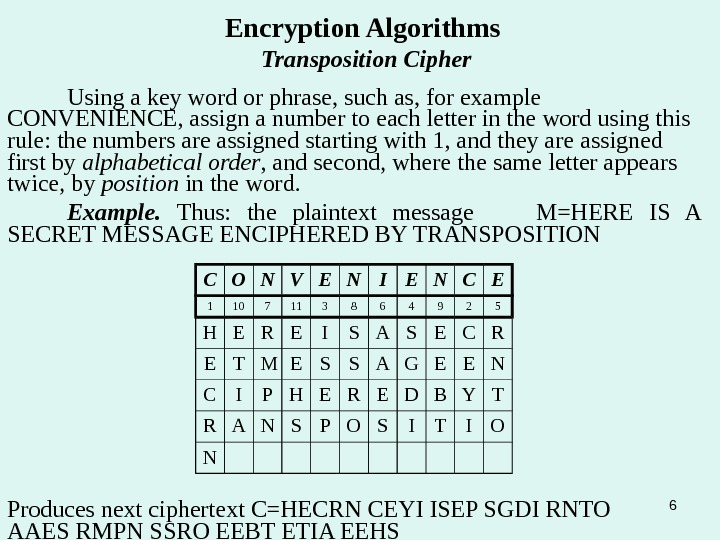6 Encryption Algorithms Transposition Cipher Using a key word or phrase, such as, for example CONVENIENCE, assign a number to each letter in the word using this rule: the numbers are assigned starting with 1, and they are assigned first by alphabetical order , and second, where the same letter appears twice, by position in the word. Example. Thus: the plaintext message M=HERE IS A SECRET MESSAGE ENCIPHERED BY TRANSPOSITION Produces next ciphertext C=HECRN CEYI ISEP SGDI RNTO AAES RMPN SSRO EEBT ETIA EEHS C O N V E N I E N C E 1 10 7 11 3 8 6 4 9 2 5 H E R E I S A S E C R E T M E S S A G E E N C I P H E R E D B Y T R A N S P O S I T I O N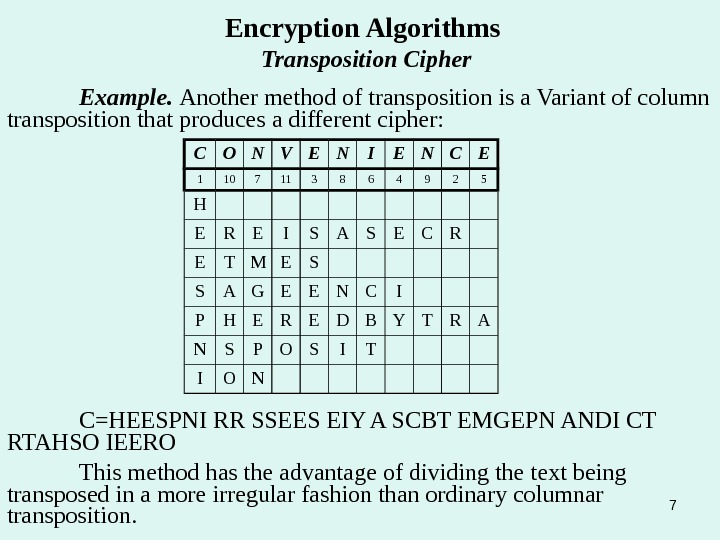7 Encryption Algorithms Transposition Cipher Example. Another method of transposition is a Variant of column transposition that produces a different cipher: C O N V E N I E N C E 1 10 7 11 3 8 6 4 9 2 5 H E R E I S A S E C R E T M E S S A G E E N C I P H E R E D B Y T R A N S P O S I T I O N C=HEESPNI RR SSEES EIY A SCBT EMGEPN ANDI CT RTAHSO IEERO This method has the advantage of dividing the text being transposed in a more irregular fashion than ordinary columnar transposition.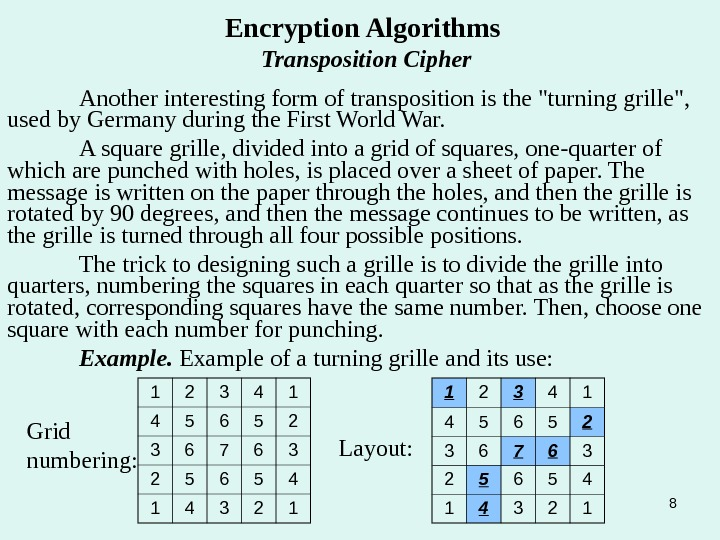8 Encryption Algorithms Transposition Cipher Another interesting form of transposition is the «turning grille», used by Germany during the First World War. A square grille, divided into a grid of squares, one-quarter of which are punched with holes, is placed over a sheet of paper. The message is written on the paper through the holes, and then the grille is rotated by 90 degrees, and then the message continues to be written, as the grille is turned through all four possible positions. The trick to designing such a grille is to divide the grille into quarters, numbering the squares in each quarter so that as the grille is rotated, corresponding squares have the same number. Then, choose one square with each number for punching. Example of a turning grille and its use: 1 2 3 4 1 4 5 6 5 2 3 6 7 6 3 2 5 6 5 4 1 4 3 2 1 Layout: Grid numbering: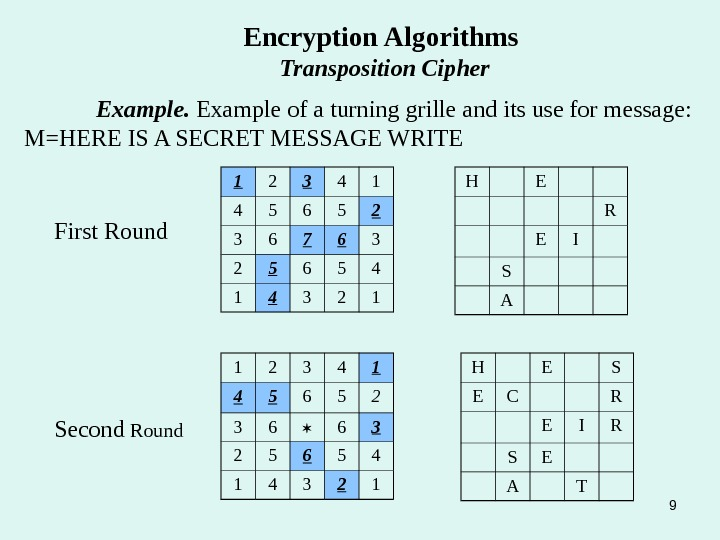91 2 3 4 1 4 5 6 5 2 3 6 7 6 3 2 5 6 5 4 1 4 3 2 1 Encryption Algorithms Transposition Cipher Example of a turning grille and its use for message: M=HERE IS A SECRET MESSAGE WRITE 1 2 3 4 1 4 5 6 5 2 3 6 6 3 2 5 6 5 4 1 4 3 2 1 H E R E I S A H E S E C R E I R S E A TFirst Round Second Round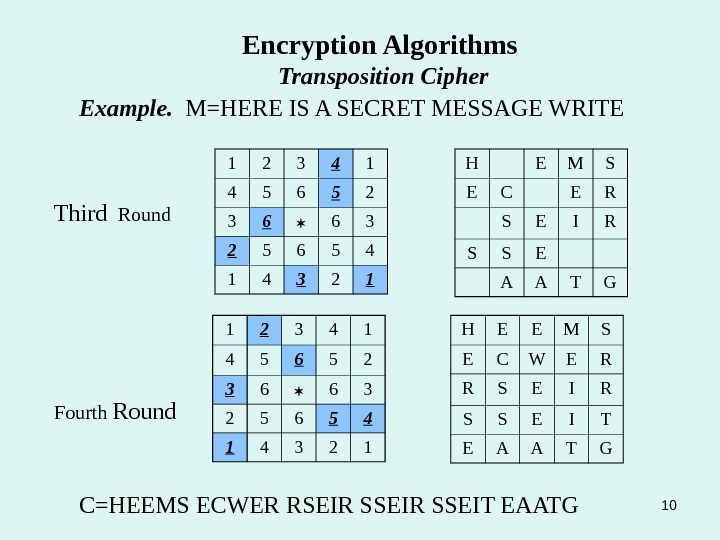101 2 3 4 1 4 5 6 5 2 3 6 6 3 2 5 6 5 4 1 4 3 2 1 Encryption Algorithms Transposition Cipher Example. M=HERE IS A SECRET MESSAGE WRITE 1 2 3 4 1 4 5 6 5 2 3 6 6 3 2 5 6 5 4 1 4 3 2 1 H E M S E C E R S E I R S S E A A T GThird Round Fourth Round H E E M S E C W E R R S E I R S S E I T E A A T G C=HEEMS ECWER RSEIR SSEIT EAATG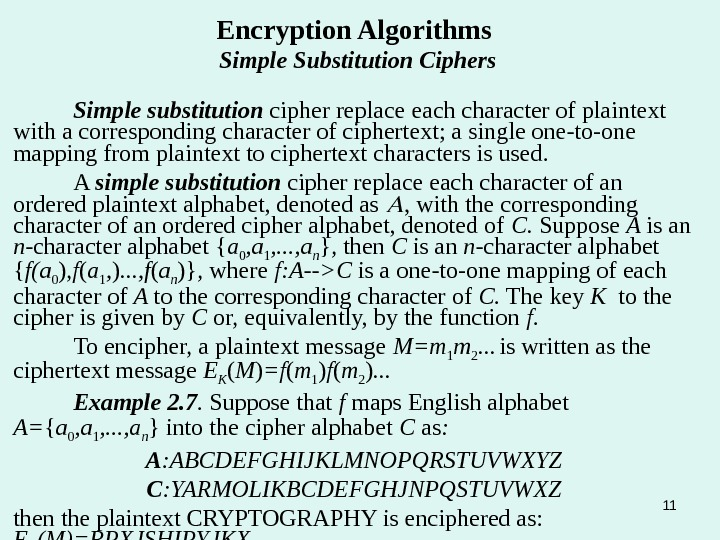11 Encryption Algorithms Simple Substitution Ciphers Simple substitution cipher replace each character of plaintext with a corresponding character of ciphertext; a single one-to-one mapping from plaintext to ciphertext characters is used. A simple substitution cipher replace each character of an ordered plaintext alphabet, denoted as , with the corresponding character of an ordered cipher alphabet, denoted of C. Suppose A is an n -character alphabet { a 0 , a 1 , . . . , a n } , then C is an n -character alphabet { f(a 0 ), f ( a 1 , ). . . , f ( a n )} , where f: A—>C is a one-to-one mapping of each character of A to the corresponding character of C. The key K to the cipher is given by C or, equivalently, by the function f. To encipher, a plaintext message M=m 1 m 2. . . is written as the ciphertext message E K ( M ) =f ( m 1 ) f ( m 2 ). . . Example 2. 7. Suppose that f maps English alphabet A= { a 0 , a 1 , . . . , a n } into the cipher alphabet C as : ABCDEFGHIJKLMNOPQRSTUVWXYZ C : YARMOLIKBCDEFGHJNPQSTUVWXZ then the plaintext CRYPTOGRAPHY is enciphered as: E K (M)=RPXJSHIPYJKX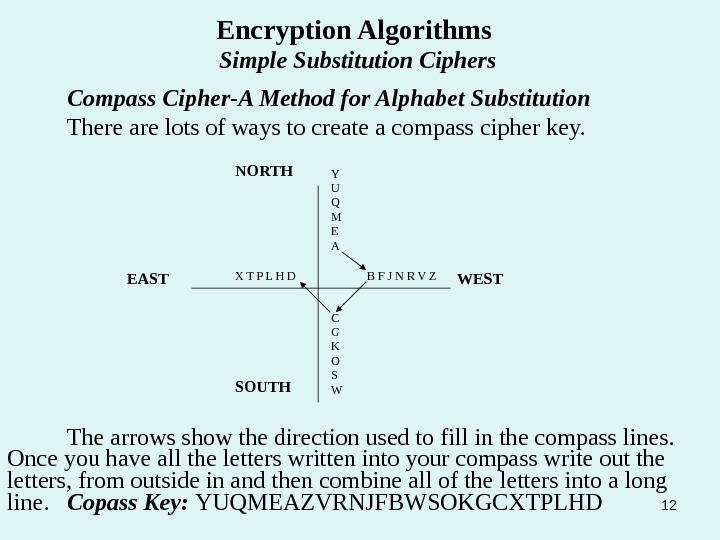12 Encryption Algorithms Simple Substitution Ciphers Compass Cipher-A Method for Alphabet Substitution There are lots of ways to create a compass cipher key. Y U Q M E A C G K O S W B F J N R V Z X T P L H D NORTH SOUTHEAST WEST The arrows show the direction used to fill in the compass lines. Once you have all the letters written into your compass write out the letters, from outside in and then combine all of the letters into a long line. Copass Key: YUQMEAZVRNJFBWSOKGCXTPLH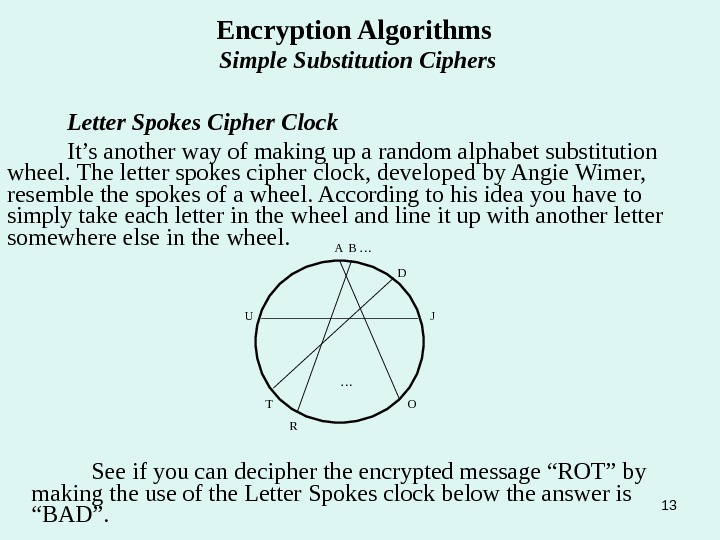13 Encryption Algorithms Simple Substitution Ciphers Letter Spokes Cipher Clock It’s another way of making up a random alphabet substitution wheel. The letter spokes cipher clock, developed by Angie Wimer, resemble the spokes of a wheel. According to his idea you have to simply take each letter in the wheel and line it up with another letter somewhere else in the wheel. A B … D U J … T O R See if you can decipher the encrypted message “ROT” by making the use of the Letter Spokes clock below the answer is “BAD”.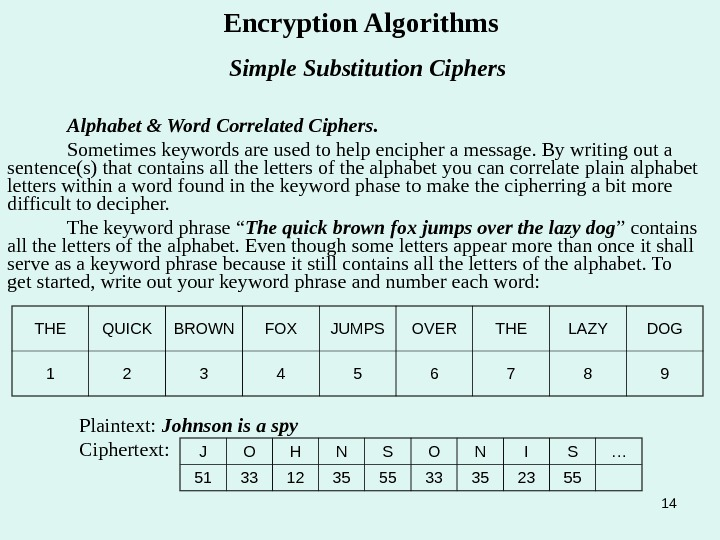14 Encryption Algorithms Simple Substitution Ciphers Alphabet & Word Correlated Ciphers. Sometimes keywords are used to help encipher a message. By writing out a sentence(s) that contains all the letters of the alphabet you can correlate plain alphabet letters within a word found in the keyword phase to make the cipherring a bit more difficult to decipher. The keyword phrase “ The quick brown fox jumps over the lazy dog ” contains all the letters of the alphabet. Even though some letters appear more than once it shall serve as a keyword phrase because it still contains all the letters of the alphabet. To get started, write out your keyword phrase and number each word: THE QUICK BROWN FOX JUMPS OVER THE LAZY DOG 1 2 3 4 5 6 7 8 9 Plaintext: Johnson is a spy Ciphertext: J O H N S O N I S …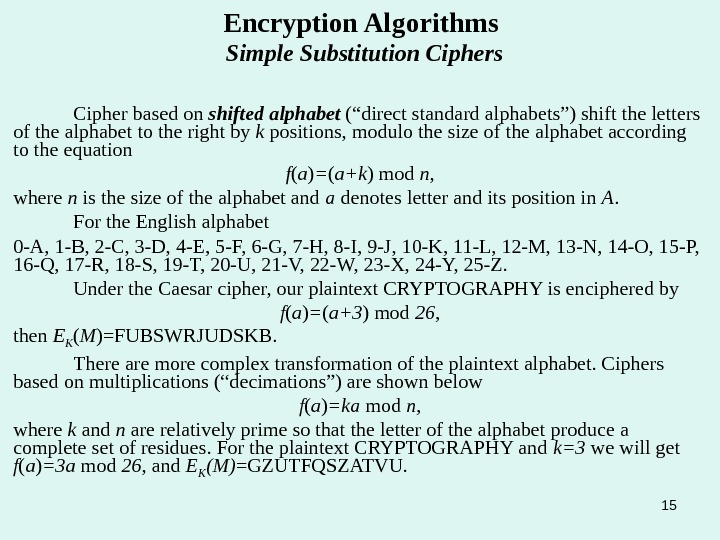15 Encryption Algorithms Simple Substitution Ciphers Cipher based on shifted alphabet (“direct standard alphabets”) shift the letters of the alphabet to the right by k positions, modulo the size of the alphabet according to the equation f ( a ) = ( a+k ) mod n, where n is the size of the alphabet and a denotes letter and its position in A. For the English alphabet 0 -A, 1 -B, 2 -C, 3 -D, 4 -E, 5 -F, 6 -G, 7 -H, 8 -I, 9 -J, 10 -K, 11 -L, 12 -M, 13 -N, 14 -O, 15 -P, 16 -Q, 17 -R, 18 -S, 19 -T, 20 -U, 21 -V, 22 -W, 23 -X, 24 -Y, 25 -Z. Under the Caesar cipher, our plaintext CRYPTOGRAPHY is enciphered by f ( a ) = ( a+3 ) mod 26, then E K ( M )=FUBSWRJUDSKB. There are more complex transformation of the plaintext alphabet. Ciphers based on multiplications (“decimations”) are shown below f ( a ) =ka mod n, where k and n are relatively prime so that the letter of the alphabet produce a complete set of residues. For the plaintext CRYPTOGRAPHY and k=3 we will get f ( a ) =3 a mod 26, and E K (M) =GZUTFQSZATVU.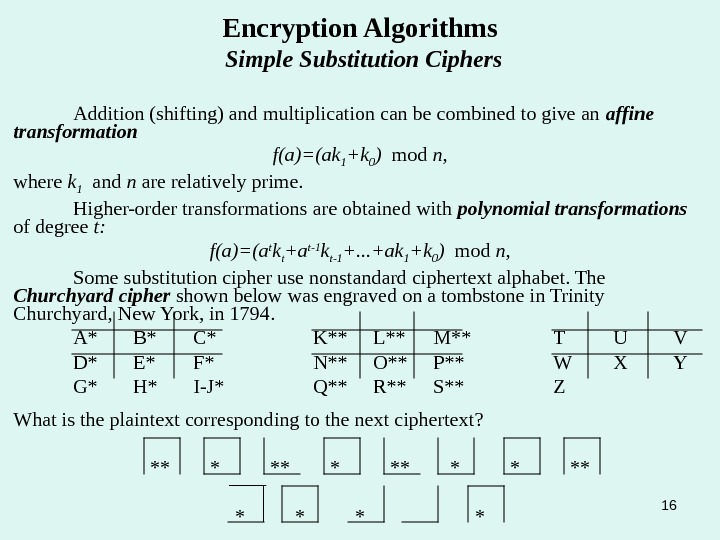16 Encryption Algorithms Simple Substitution Ciphers Addition (shifting) and multiplication can be combined to give an affine transformation f(a)=(ak 1 +k 0 ) mod n, where k 1 and n are relatively prime. Higher-order transformations are obtained with polynomial transformations of degree t: f(a)=(a t k t +a t-1 k t-1 +. . . +ak 1 +k 0 ) mod n, Some substitution cipher use nonstandard ciphertext alphabet. The Churchyard cipher shown below was engraved on a tombstone in Trinity Churchyard, New York, in 1794. A* B* C* K** L* M** T U V D* E* F* N** O* P** W X Y G* H* I-J* Q** R** S** Z What is the plaintext corresponding to the next ciphertext? ** * * * *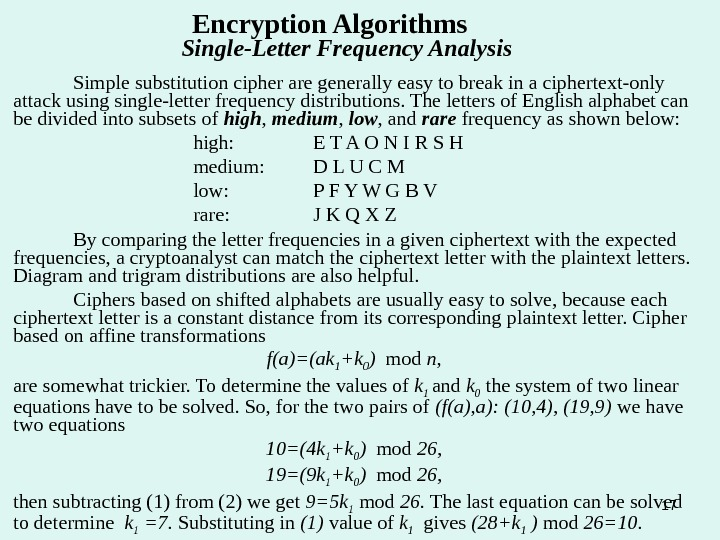17 Encryption Algorithms Single-Letter Frequency Analysis Simple substitution cipher are generally easy to break in a ciphertext-only attack usingle-letter frequency distributions. The letters of English alphabet can be divided into subsets of high , medium , low , and rare frequency as shown below: high: E T A O N I R S H medium: D L U C M low: P F Y W G B V rare: J K Q X Z By comparing the letter frequencies in a given ciphertext with the expected frequencies, a cryptoanalyst can match the ciphertext letter with the plaintext letters. Diagram and trigram distributions are also helpful. Ciphers based on shifted alphabets are usually easy to solve, because each ciphertext letter is a constant distance from its corresponding plaintext letter. Cipher based on affine transformations f(a)=(ak 1 +k 0 ) mod n, are somewhat trickier. To determine the values of k 1 and k 0 the system of two linear equations have to be solved. So, for the two pairs of (f(a), a): (10, 4), (19, 9) we have two equations 10=(4 k 1 +k 0 ) mod 26, 19=(9 k 1 +k 0 ) mod 26, then subtracting (1) from (2) we get 9=5 k 1 mod 26. The last equation can be solved to determine k 1 =7. Substituting in (1) value of k 1 gives (28+k 1 ) mod 26=10.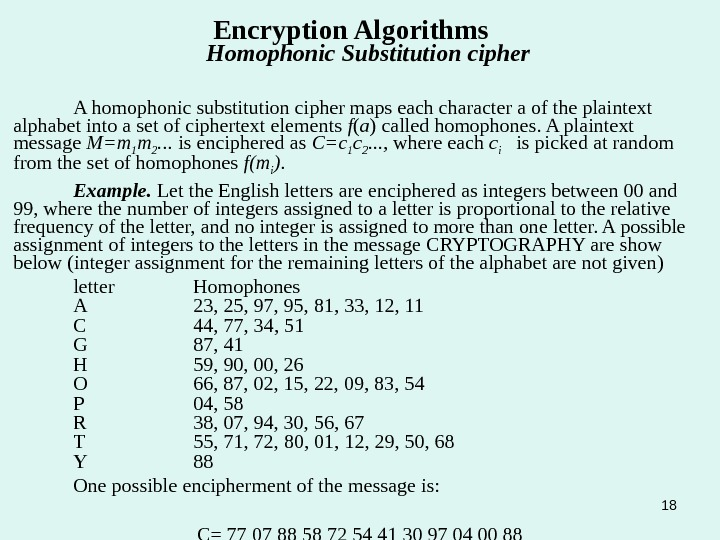18 Encryption Algorithms Homophonic Substitution cipher A homophonic substitution cipher maps each character a of the plaintext alphabet into a set of ciphertext elements f ( a ) called homophones. A plaintext message M=m 1 m 2. . . is enciphered as C=c 1 c 2. . . , where each c i is picked at random from the set of homophones f(m i ). Example. Let the English letters are enciphered as integers between 00 and 99, where the number of integers assigned to a letter is proportional to the relative frequency of the letter, and no integer is assigned to more than one letter. A possible assignment of integers to the letters in the message CRYPTOGRAPHY are show below (integer assignment for the remaining letters of the alphabet are not given) letter Homophones A 23, 25, 97, 95, 81, 33, 12, 11 C 44, 77, 34, 51 G 87, 41 H 59, 90, 00, 26 O 66, 87, 02, 15, 22, 09, 83, 54 P 04, 58 R 38, 07, 94, 30, 56, 67 T 55, 71, 72, 80, 01, 12, 29, 50, 68 Y 88 One possible encipherment of the message is: C=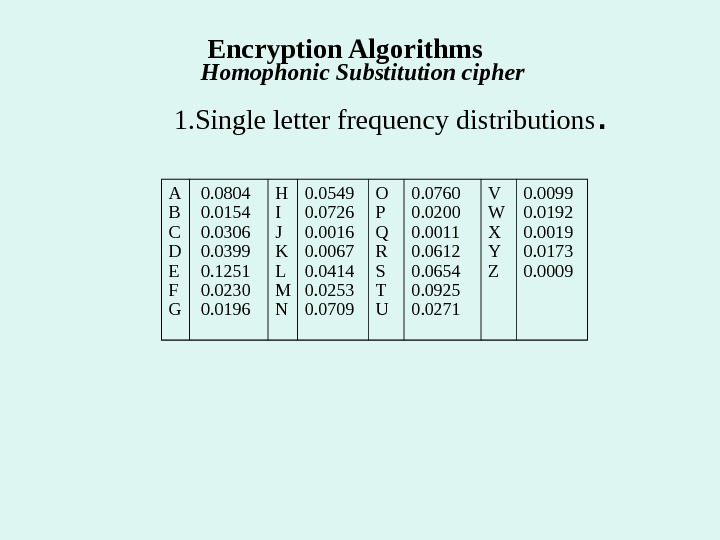1. Single letter frequency distributions. A B C D E F G 0. 0804 0. 0154 0. 0306 0. 0399 0. 1251 0. 0230 0. 0196 H I J K L M N 0. 0549 0. 0726 0. 0016 0. 0067 0. 0414 0. 0253 0. 0709 O P Q R S T U 0. 0760 0. 0200 0. 0011 0. 0612 0. 0654 0. 0925 0. 0271 V W X Y Z 0. 0099 0. 0192 0. 0019 0. 0173 0. 0009 Encryption Algorithms Homophonic Substitution cipher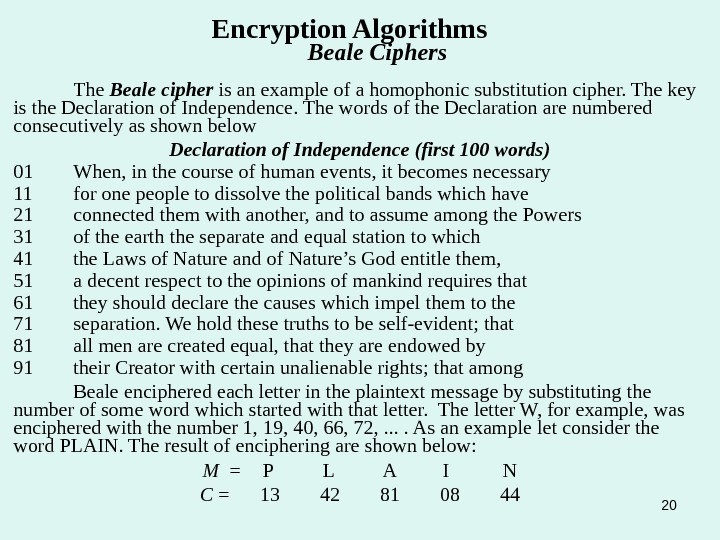20 Encryption Algorithms Beale Ciphers The Beale cipher is an example of a homophonic substitution cipher. The key is the Declaration of Independence. The words of the Declaration are numbered consecutively as shown below Declaration of Independence (first 100 words) 01 When, in the course of human events, it becomes necessary 11 for one people to dissolve the political bands which have 21 connected them with another, and to assume among the Powers 31 of the earth the separate and equal station to which 41 the Laws of Nature and of Nature’s God entitle them, 51 a decent respect to the opinions of mankind requires that 61 they should declare the causes which impel them to the 71 separation. We hold these truths to be self-evident; that 81 all men are created equal, that they are endowed by 91 their Creator with certain unalienable rights; that among Beale enciphered each letter in the plaintext message by substituting the number of some word which started with that letter. The letter W, for example, was enciphered with the number 1, 19, 40, 66, 72, . . As an example let consider the word PLAIN. The result of enciphering are shown below: M = P L A I N C =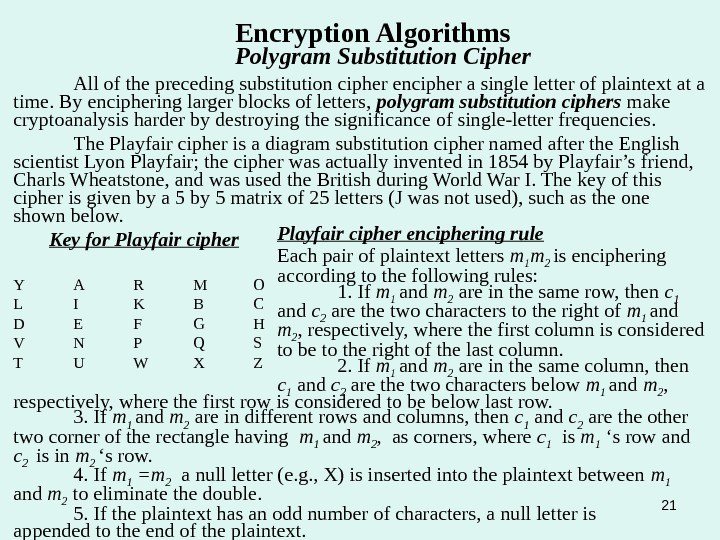21 Encryption Algorithms Polygram Substitution Cipher All of the preceding substitution cipher encipher a single letter of plaintext at a time. By enciphering larger blocks of letters, polygram substitution ciphers make cryptoanalysis harder by destroying the significance of single-letter frequencies. The Playfair cipher is a diagram substitution cipher named after the English scientist Lyon Playfair; the cipher was actually invented in 1854 by Playfair’s friend, Charls Wheatstone, and was used the British during World War I. The key of this cipher is given by a 5 by 5 matrix of 25 letters (J was not used), such as the one shown below. Key for Playfair cipher Y A R M O L I K B C D E F G H V N P Q S T U W X Z Playfair cipher enciphering rule Each pair of plaintext letters m 1 m 2 is enciphering according to the following rules: 1. If m 1 and m 2 are in the same row, then c 1 and c 2 are the two characters to the right of m 1 and m 2 , respectively, where the first column is considered to be to the right of the last column. 2. If m 1 and m 2 are in the same column, then c 1 and c 2 are the two characters below m 1 and m 2 , respectively, where the first row is considered to be below last row. 3. If m 1 and m 2 are in different rows and columns, then c 1 and c 2 are the other two corner of the rectangle having m 1 and m 2 , as corners, where c 1 is m 1 ‘s row and c 2 is in m 2 ‘s row. 4. If m 1 =m 2 a null letter (e. g. , X) is inserted into the plaintext between m 1 and m 2 to eliminate the double. 5. If the plaintext has an odd number of characters, a null letter is appended to the end of the plaintext.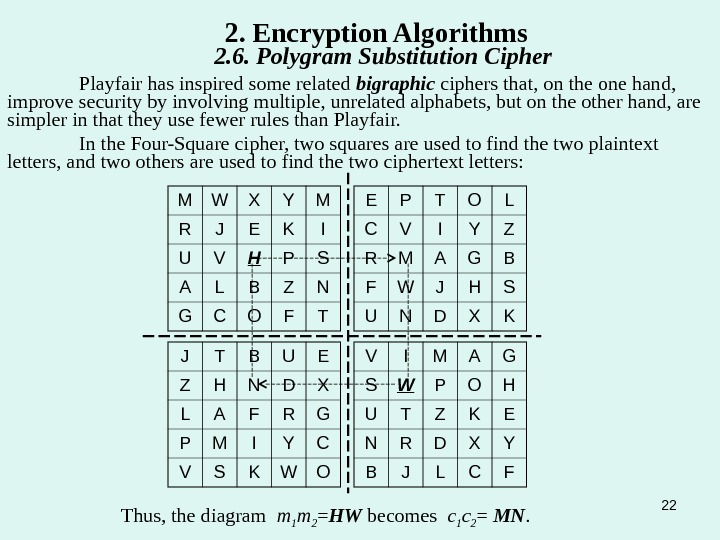22 Playfair has inspired some related bigraphic ciphers that, on the one hand, improve security by involving multiple, unrelated alphabets, but on the other hand, are simpler in that they use fewer rules than Playfair. In the Four-Square cipher, two squares are used to find the two plaintext letters, and two others are used to find the two ciphertext letters: 2. Encryption Algorithms 2. 6. Polygram Substitution Cipher M W X Y M R J E K I U V H P S A L B Z N G C O F T V I M A G S W P O H U T Z K E N R D X Y B J L C FJ T B U E Z H N D X L A F R G P M I Y C V S K W O E P T O L C V I Y Z R M A G B F W J H S U N D X K Thus, the diagram m 1 m 2 = HW becomes c 1 c 2 = MN.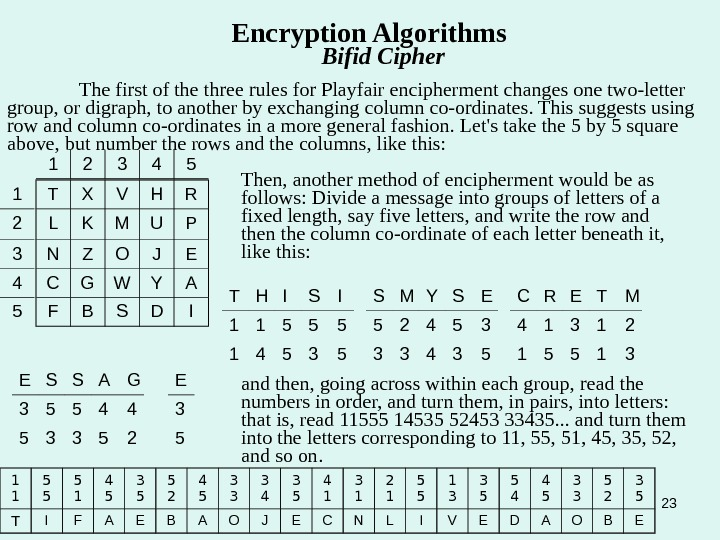23 The first of the three rules for Playfair encipherment changes one two-letter group, or digraph, to another by exchanging column co-ordinates. This suggests using row and column co-ordinates in a more general fashion. Let’s take the 5 by 5 square above, but number the rows and the columns, like this: Encryption Algorithms Bifid Cipher T X V H R L K M U P N Z O J E C G W Y A F B S D I 1 2 3 4 5 Then, another method of encipherment would be as follows: Divide a message into groups of letters of a fixed length, say five letters, and write the row and then the column co-ordinate of each letter beneath it, like this: T H I S I 1 1 5 5 5 1 4 5 3 5 C R E T M 4 1 3 1 2 1 5 5 1 3 E S S A G 3 5 5 4 4 5 3 3 5 2 S M Y S E 5 2 4 5 3 3 3 4 3 5 E 3 5 and then, going across within each group, read the numbers in order, and turn them, in pairs, into letters: that is, read 11555 14535 52453 33435. . . and turn them into the letters corresponding to 11, 55, 51, 45, 35, 52, and so on. 1 1 5 5 5 1 5 3 5 5 2 5 3 3 5 1 3 1 2 1 5 5 1 3 3 5 5 5 3 3 5 2 3 5 T I F A E B A O J E C N L I V E D A O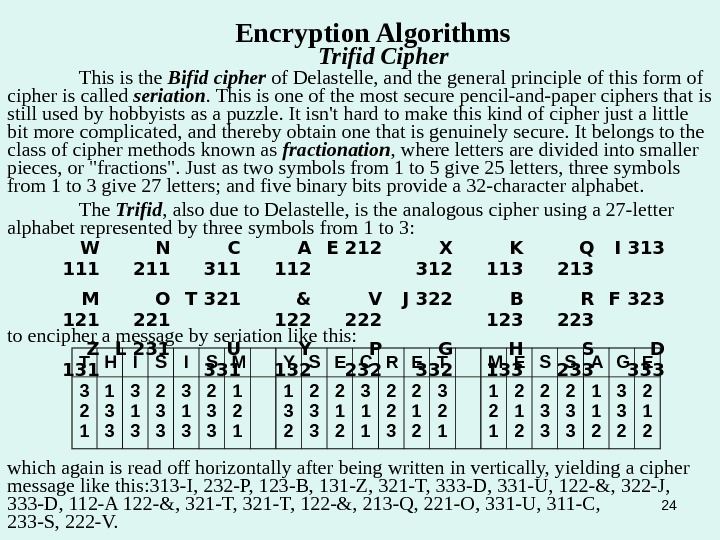24 Encryption Algorithms Trifid Cipher This is the Bifid cipher of Delastelle, and the general principle of this form of cipher is called seriation. This is one of the most secure pencil-and-paper ciphers that is still used by hobbyists as a puzzle. It isn’t hard to make this kind of cipher just a little bit more complicated, and thereby obtain one that is genuinely secure. It belongs to the class of cipher methods known as fractionation , where letters are divided into smaller pieces, or «fractions». Just as two symbols from 1 to 5 give 25 letters, three symbols from 1 to 3 give 27 letters; and five binary bits provide a 32 -character alphabet. The Trifid , also due to Delastelle, is the analogous cipher using a 27 -letter alphabet represented by three symbols from 1 to 3: W 111 N 211 C 311 A 112 E 212 X 312 K 113 Q 213 I 313 M 121 O 221 T 321 & 122 V 222 J 322 B 123 R 223 F 323 Z 131 L 231 U 331 Y 132 P 232 G 332 H 133 S 233 D 333 to encipher a message by seriation like this: T H I S M Y S E C R E T M E S S A G E 3 2 1 1 3 3 3 1 3 2 3 3 1 2 1 1 3 2 2 3 3 2 1 2 3 1 1 2 2 3 2 1 1 2 1 2 2 3 3 1 1 2 3 3 2 2 1 2 which again is read off horizontally after being written in vertically, yielding a cipher message like this: 313 -I, 232 -P, 123 -B, 131 -Z, 321 -T, 333 -D, 331 -U, 122 -&, 322 -J, 333 -D, 112 -A 122 -&, 321 -T, 122 -&, 213 -Q, 221 -O, 331 -U, 311 -C, 233 -S, 222 -V.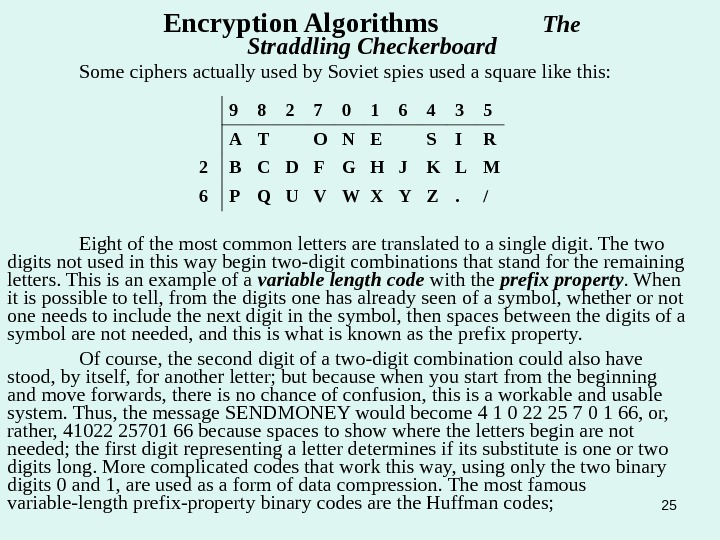25 Some ciphers actually used by Soviet spies used a square like this: Encryption Algorithms The Straddling Checkerboard 9 8 2 7 0 1 6 4 3 5 A T O N E S I R 2 B C D F G H J K L M 6 P Q U V W X Y Z. / Eight of the most common letters are translated to a single digit. The two digits not used in this way begin two-digit combinations that stand for the remaining letters. This is an example of a variable length code with the prefix property. When it is possible to tell, from the digits one has already seen of a symbol, whether or not one needs to include the next digit in the symbol, then spaces between the digits of a symbol are not needed, and this is what is known as the prefix property. Of course, the second digit of a two-digit combination could also have stood, by itself, for another letter; but because when you start from the beginning and move forwards, there is no chance of confusion, this is a workable and usable system. Thus, the message SENDMONEY would become 4 1 0 22 25 7 0 1 66, or, rather, 41022 25701 66 because spaces to show where the letters begin are not needed; the first digit representing a letter determines if its substitute is one or two digits long. More complicated codes that work this way, using only the two binary digits 0 and 1, are used as a form of data compression. The most famous variable-length prefix-property binary codes are the Huffman codes;